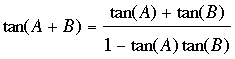SEARCH HOMEMath Central Quandaries & QueriesQuestion from Evan, a student: Could you please help me with this question... Derive an expression for tan3x in terms of tanxEvan,

I assume that you know the fact thatWrite tan(3x) as tan(2x + x) and then use the expression above to write tan(3x) in terms of tan(x) and tan(2x). Next write tan(2x) as tan(x + x) and use the expression above again to write tan(3x) in terms of tan(x). Simplify the expression.

HarleyMath Central is supported by the University of Regina and The Pacific Institute for the Mathematical Sciences.